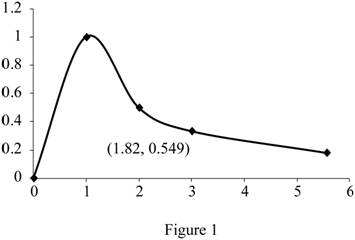# The average value of the function f ( x ) = 1 x .### Single Variable Calculus: Concepts...

4th Edition
James Stewart
Publisher: Cengage Learning
ISBN: 9781337687805### Single Variable Calculus: Concepts...

4th Edition
James Stewart
Publisher: Cengage Learning
ISBN: 9781337687805

#### Solutions

Chapter 6.5, Problem 8E

(a)

To determine

## To find: The average value of the function f(x)=1x.

Expert Solution

The average value of the function is 0.549.

### Explanation of Solution

Given information:

The function is f(x)=1x.

Calculation:

Show the function as follows:

f(x)=1x (1)

The expression to find the average value of the function (favg) is shown below:

favg=1baabf(x)dx (2)

Here, the upper limit is b, the lower limit is a, and the function is f(x).

Substitute 1 for a, 3 for b, and 1x for f(x) in Equation (2).

fave=131131xdx (3)

Integrate Equation (3) with respect to x and apply the limits.

fave=12[lnx]13=12[ln3ln1]=12[1.0986]=0.549

Thus, the average value of the function is 0.549.

(b)

To determine

### To find: The value of count c.

Expert Solution

The value of c is 1.82.

### Explanation of Solution

Given information:

The function is f(x)=1x.

Calculation:

Find the value of c using the formula:

f(c)=favg (4)

Substitute 0.549 for fave in Equation (4).

f(c)=0.549 (5)

Substitute c for x in Equation (1)

f(c)=1c (6)

Substitute 0.549 for f(c) in Equation (5)

0.549=1cc=1.82

Thus, the value of c is 1.82.

(c)

To determine

Expert Solution

### Explanation of Solution

Substitute 1.82 for x in Equation (1)

y=1x=11.82=0.549

Draw the graph for the curve function y=1x and mark the coordinates (1.82,0.549) as shown in Figure 1.### Have a homework question?

Subscribe to bartleby learn! Ask subject matter experts 30 homework questions each month. Plus, you’ll have access to millions of step-by-step textbook answers!